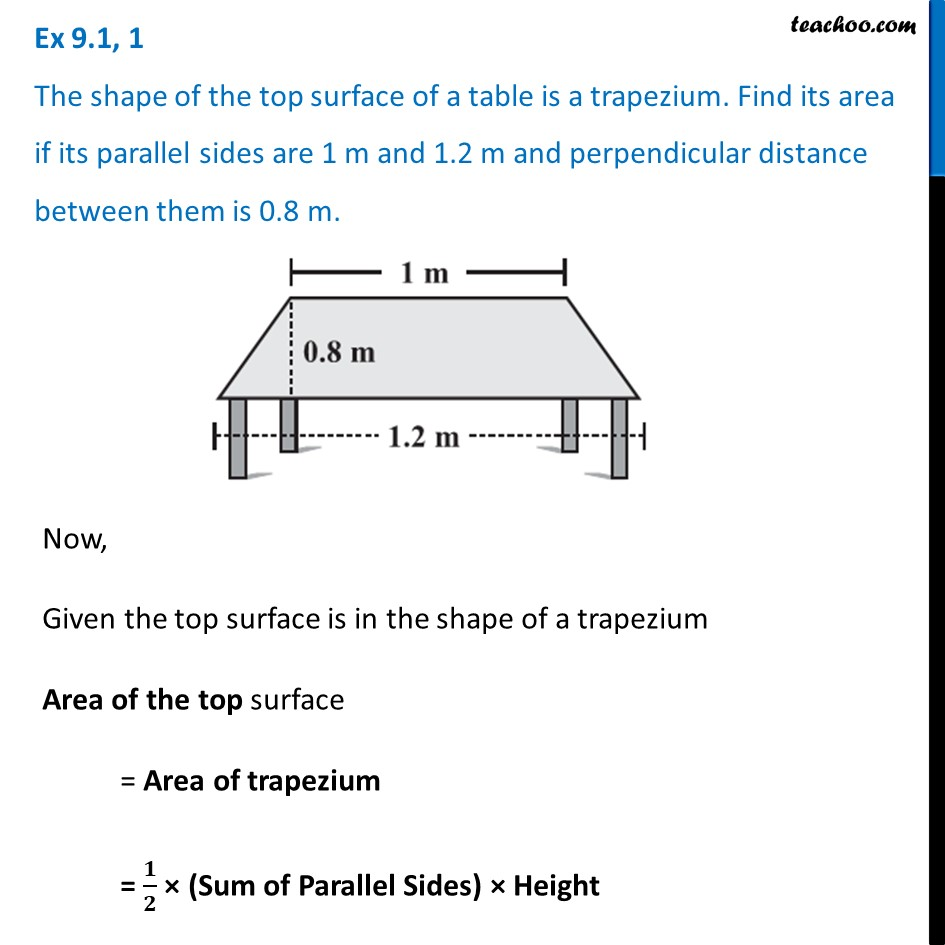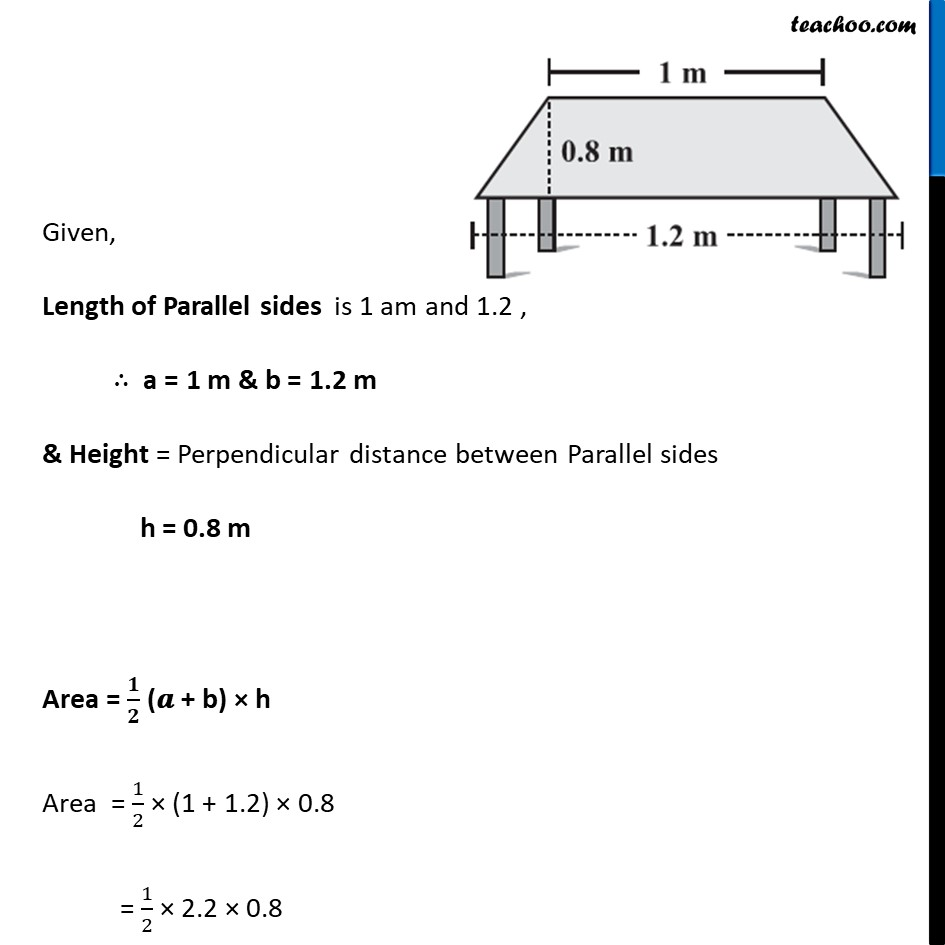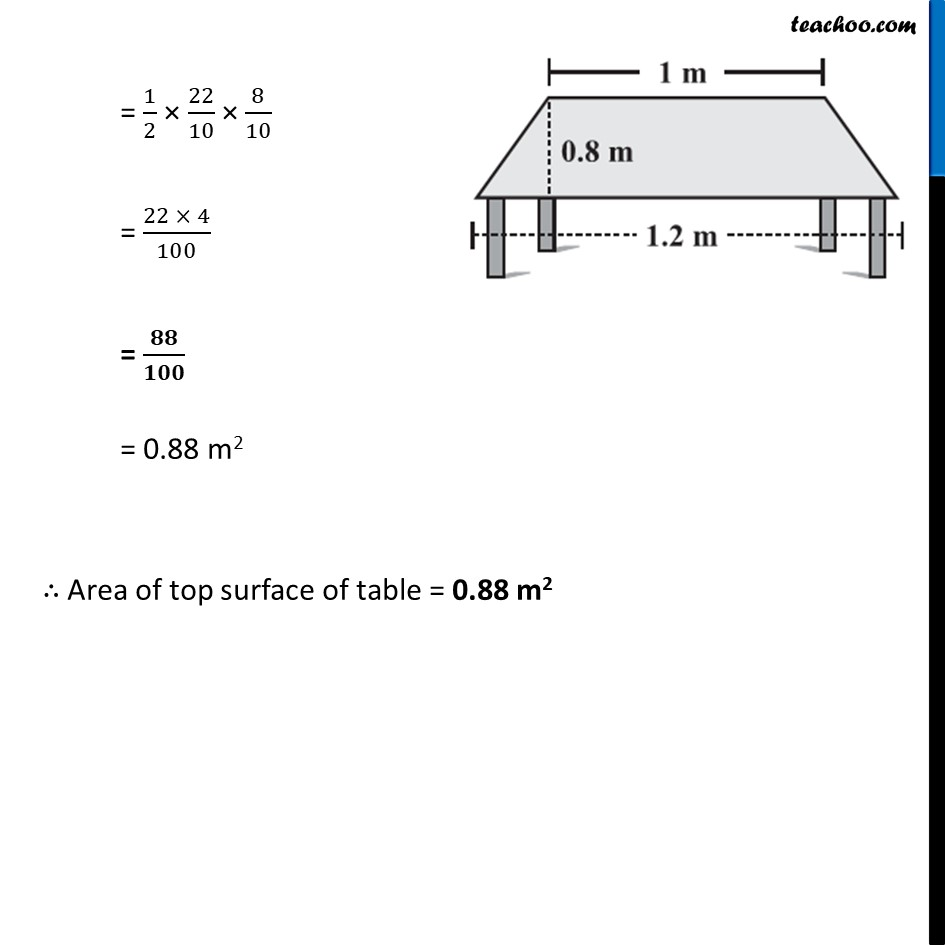Ex 9.1

Chapter 9 Class 8 Mensuration
Serial order wiseLearn in your speed, with individual attention - Teachoo Maths 1-on-1 Class

### Transcript

Ex 9.1, 1 The shape of the top surface of a table is a trapezium. Find its area if its parallel sides are 1 m and 1.2 m and perpendicular distance between them is 0.8 m. Now, Given the top surface is in the shape of a trapezium Area of the top surface = Area of trapezium = 𝟏/𝟐 × (Sum of Parallel Sides) × Height Given, Length of Parallel sides is 1 am and 1.2 , ∴ a = 1 m & b = 1.2 m & Height = Perpendicular distance between Parallel sides h = 0.8 m Area = 𝟏/𝟐 (𝒂 + b) × h Area = 1/2 × (1 + 1.2) × 0.8 = 1/2 × 2.2 × 0.8 = 1/2 × 22/10 × 8/10 = (22 × 4)/100 = 𝟖𝟖/𝟏𝟎𝟎 = 0.88 m2 ∴ Area of top surface of table = 0.88 m2